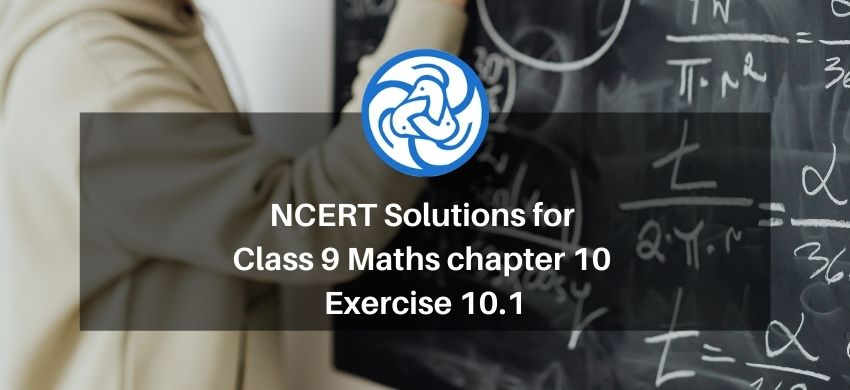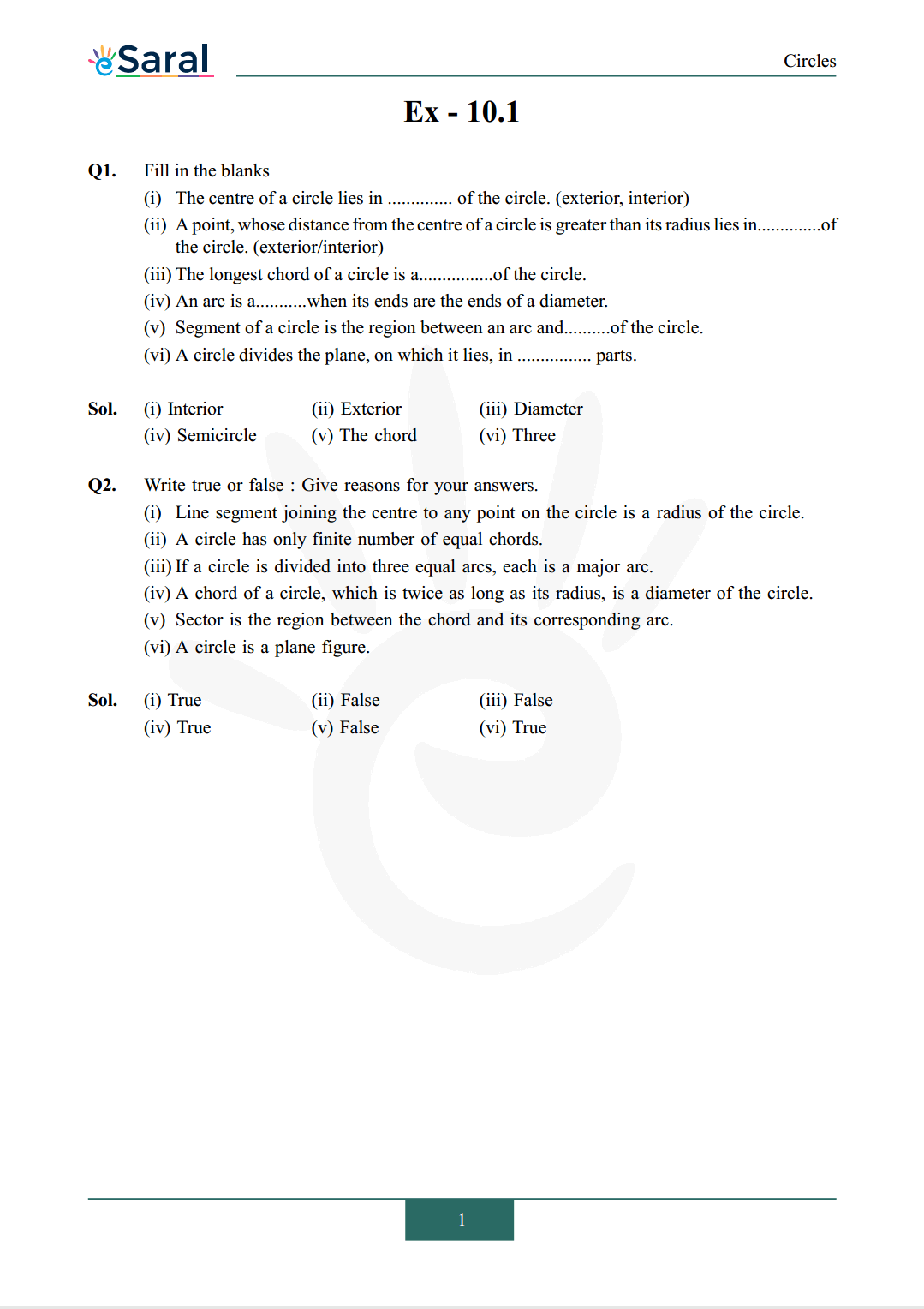Most Affordable JEE | NEET | 8,9,10 Preparation by Kota's Top IITian Doctor Faculties

# NCERT Solutions for Class 9 Maths chapter 10 Exercise 10.1 - Circles - Free PDF Download`
Hey, are you a class 9 Student and Looking for Ways to Download NCERT Solutions for Class 9 Maths chapter 10 Exercise 10.1? If Yes then you are at the right place.

Here we have listed Class 9 maths chapter 10 exercise 10.1 solutions in PDF that is prepared by Kota’s top IITian’s Faculties by keeping Simplicity in mind.

If you want to score high in your class 9 Maths Exam then it is very important for you to have a good knowledge of all the important topics, so to learn and practice those topics you can use eSaral NCERT Solutions.

So, without wasting more time Let’s start.

### Download The PDF of NCERT Solutions for Class 9 Maths chapter 10 Exercise 10.1 "Circles"#### All Questions of Chapter 10 Exercise 10.1

Once you complete the chapter 10 then you can revise Ex. 10.1 by solving following questions

Q1. Fill in the blanks
(i) The centre of a circle lies in .............. of the circle. (exterior, interior)
(ii) A point, whose distance from the centre of a circle is greater than its radius lies in..............of the circle. (exterior/interior)
(iii) The longest chord of a circle is a................of the circle.
(iv) An arc is a...........when its ends are the ends of a diameter.
(v) Segment of a circle is the region between an arc and..........of the circle.
(vi) A circle divides the plane, on which it lies, in ................ parts.

(i) Line segment joining the centre to any point on the circle is a radius of the circle.
(ii) A circle has only finite number of equal chords.
(iii) If a circle is divided into three equal arcs, each is a major arc.
(iv) A chord of a circle, which is twice as long as its radius, is a diameter of the circle.
(v) Sector is the region between the chord and its corresponding arc.
(vi) A circle is a plane figure.